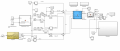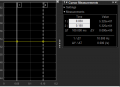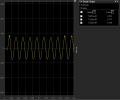# PWM signal to inverter compatible with both delta and wye connected loads

#### Øivind Bakke

Joined Aug 22, 2017
12
Greetings,

in short, my Matlab-program is suppose to control an electric machine (Not feedback-controlling) and it should be compatible with both delta and wye connected machines. (manual switch managed by the user)
At first it asks the user for a requested rms stator current. From there it transform it into dq-current and calculates the dq-voltage and does a Park and Clark inverse transformation to get the equivalent phase voltages.
Questions are as follows:
1)How should I interpret the given voltage?
-By now, I've interpreted that as the respective line-to-neutral voltage, by that I mean the voltage in each line independent of the load and the other lines.
2)If my interpretation of the voltage in question one is true then, is there a factor difference of the output voltage depending if the load connection is delta or wye?
-In my head, it shouldn't be, because the line to line voltages will be different for delta and wye because of the structure, right? Then again, by this time I've read too many articles about this topic without getting any clarification and my head can't be trusted.
3)If the voltage is straight up stator voltage, which also makes sense somehow, what's the factor difference going from delta to wye connected load?

Attached to this thread is pictures of my program to maybe help clarify exactly what my problem is. If additional clarification is needed, let me know.

Regards,
Oivind Harket Bakke1)Matlab model. Don't mind the epsilon, I only simulate. The vorsteuerwinkel is set to an arbitrary number for simulation purposes only. (Id gets a value)
2)My calculated RMS line voltage.
3)First harmonic of the first line voltage filtered out. Correspond to rms voltage of .

#### Øivind Bakke

Joined Aug 22, 2017
12
I realize my comment on the first question is poorly formulated. My problem is, that we've only regarded wye connected motors in my lectures and how we can control that with pwm and so forth. The first attachment (the model) should be valid for a wye connected motor, but is it also valid for a delta connected one? This is part of a bigger project, where my motor is a BLDC delta connected motor. I struggle finding information regarding changing the pwm and inverter depending if the load is delta or wye.

#### tcmtech

Joined Nov 4, 2013
2,867
In a typical three phase motor there is no major difference in Delta or Wye operation other than their respective voltage and current requirements for a given output.

Rather why a three phase VFD (Variable Frequency Drive) doesn't care if the motor is wired in a Delta or Wye configuration just as long as it's output matches the motors voltage and current limits for whatever connection is being used and the frequency range being applied to i the motor.